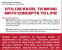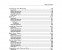### Excel for the Math Classroom PDF eBook

Written for the middle school math classroom. This books shows how to use Excel for:

• Calculation basics
• Printing grid paper
• Cartesian Coordinate Grids
• Multiplication Tables
• Math Exercise Sheets
• Arithmetic Facts Quiz
• Homework Checker
• Magic Squares
• Coordinate Grid Matching
• Math Art
• Candy Bar Fractions
• Math Facts Game
• Secret Code Maker
• Probability with Coins or Dice
• Demonstrating or Comparing Fractions with Charts
• Finding Maximum Area and Volume
• Solving a System of Equations

#### Affiliates

Like this product?

Spread the word about it and earn 20.00% of the purchase price on sales you refer.